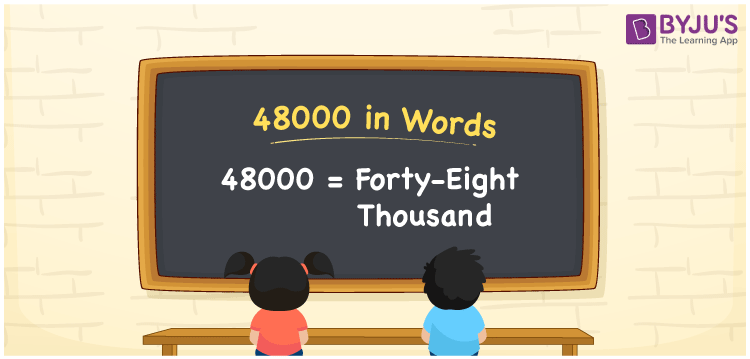# 48000 in Words

48000 in words can be written as Forty-eight thousand. We can convert 48000 into words using a place value chart easily. If you have contributed Rs. 48000 for a charity, you can say, “I contributed Forty-eight thousand rupees to a charity”. We know that 48000 is a cardinal number as it represents a specific amount.

 48000 in words Forty-eight Thousand Forty-eight Thousand in Numbers 48000

## 48000 in English Words

We generally write numbers in words with the help of the English alphabet. Therefore, we can read 48000 in English as “Forty-eight thousand”.## How to Write 48000 in Words?

As mentioned above, in this section you will learn how to write 48000 in words using a place value chart. The number 48000 contains 5 digits, so we need to make a chart that contains the place value up to 5 digits as shown below.

 Ten Thousands Thousands Hundreds Tens Ones 4 8 0 0 0

Thus, we can write the expanded form as:

4 × Ten Thousand + 8 × Thousand + 0 × Hundred + 0 × Ten + 0 × One

= 4 × 10000 + 8 × 1000 + 0 × 100 + 0 × 10 + 0 × 1

= 40000 + 8000

= 48000

= Forty-eight thousand

In the above place value chart, we see that the digits in ones, tens, hundreds are 0s. Hence, we can consider the numbers at thousand and ten thousand places, and by combining these terms, we get forty-eight thousand since 4 × 10 = 40, i.e. multiplying ten by 4, we get forty and 40 + 8 = 48, i.e. forty-eight.

Therefore, 48000 in words is written as Forty-eight thousand.

48000 is a natural number that precedes 48001 and succeeds 47999.

48000 in words – Forty-eight thousand

Is 48000 an odd number? – No

Is 48000 an even number? – Yes

Is 48000 a perfect square number? – No

Is 48000 a perfect cube number? – No

Is 48000 a prime number? – No

Is 48000 a composite number? – Yes

## Frequently Asked Questions on 48000 in Words

Q1

### How do you write 48000 in terms of words in English?

We can write the number 48000 in English words as “Forty-eight thousand”.
Q2

### What is the spelling of 48000 in words?

The spelling of 48000 is used to pronounce the number, which is forty-eight thousand.
Q3

### How do you write 48000 in words on a cheque?

On a cheque, we should write 48000 in words as Forty-eight Thousand Rupees only.